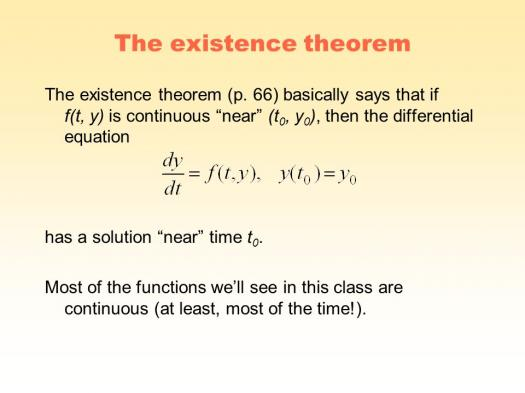# Existence Theorem Assessment Test

10 Questions | Total Attempts: 114SettingsExistence theorems are mathematical theorems with a statement that begins with 'there exist(s). . '. It is a theorem with a prenex normal form involving the existential quantifier. Take this test to find out more.

• 1.
Find the differential dy of the given function. y= 3x 2 - 4
• A.

40x dx

• B.

12x dx

• C.

6x dx

• D.

36x dx

• 2.
Find the limit. lim(4 + 3/x) x->∞
• A.

1

• B.

2

• C.

3

• D.

4

• 3.
The Rolle's Theorem is named after who?
• A.

Michel Rolle

• B.

Janet Rolle

• C.

Albert Rolle

• D.

Pierce Rolle

• 4.
Find any critical numbers of the function. f(x)= x 3 - 3x 2
• A.

X=1 , x=2

• B.

X=0 , x =3

• C.

X=1 , x=3

• D.

X=0 , x=2

• 5.
If there is an open interval containing c on which f(c) is a maximun, then f(c) is called...
• A.

Absolute minimum

• B.

Relative maximum

• C.

Absolute maximum

• D.

Relative minimum

• 6.
If f is continuous on a closed interval [a,b], then f has both a minimum and a maximum on the interval.
• A.

The mean value theorem

• B.

Pythagoraean theorem

• C.

Binomial theorem

• D.

The extreme value theorem

• 7.
What is the average rate of change of the function below over the indicated interval? f(x) = x2 - 3x - 28, [-4,7]
• A.

11

• B.

66

• C.

6

• D.

0

• 8.
What is the average rate of change of the function below over the indicated interval? f(x) = 12√(x), [1,16]?
• A.

12/5

• B.

0

• C.

1

• D.

24

• 9.
What is x-value at which the function below has the same slope as the average rate of change over the indicated interval? f(x) = 12√(x), [1,16]?
• A.

24

• B.

18.78

• C.

6.25

• D.

7.35

• 10.
The graph of f is concave upward or downward on I if f ' is increasing on the interval...
• A.

Concave upwards

• B.

Convex upwards

• C.

Concave downwards

• D.

Convex downwards

Related TopicsBack to top